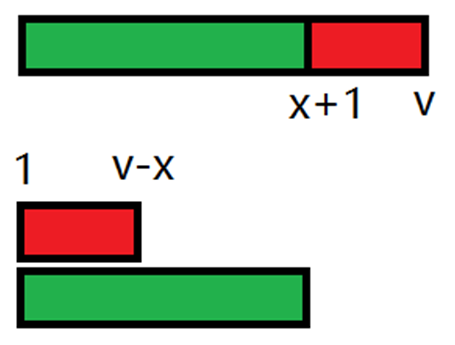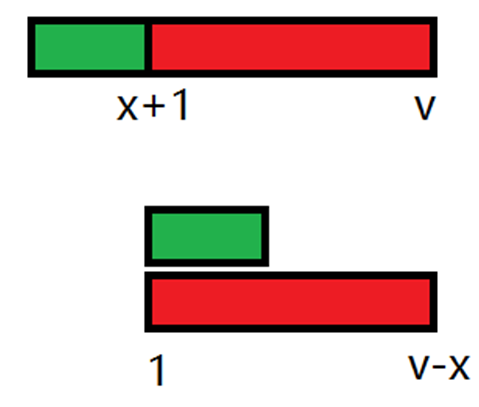# 博客

## [Ynoi2018]Day1题解

2018-12-12 16:09:11 By OldDriverTree

### T1：いろとりどりのセカイ（Version3）

Idea：lxl Solution：lxl Std：lxl Data：lxl

https://www.luogu.org/problemnew/solution/P4117

https://www.lydsy.com/JudgeOnline/problem.php?id=5143

http://codeforces.com/problemset/problem/896/E

"突刺贯穿的第二分块" （妈呀这名字好中二啊（谁叫我要用日本轻小说中的东西命名真是作死））$O( 1 )$把块内值为x的全部变成$x+v$

$O( \sqrt n )$求出块内经过大量操作后，每个数的值

1.定义f[i][j]为第i块里面所有值为j的数的下标的链表

2.可以每块每个值维护一个vector

3.可以用一个并查集维护

1. merge( x , y )

2. for( i = 1 ; i <= sqrtn ; i++ ) find( i )### T2：終末なにしてますか？忙しいですか？救ってもらっていいですか？（Version6）

Idea：lxl&zyz Solution：ccz Std：lxl Data：lxl

https://www.luogu.org/problemnew/show/P4680

https://www.lydsy.com/JudgeOnline/problem.php?id=5144

"深浅值藏的第六分块" （妈呀这名字好中二啊（谁叫我要用日本轻小说中的东西命名真是作死））

https://www.lydsy.com/JudgeOnline/problem.php?id=5089

cur -> L()为cur节点内部的左端最大前缀和的一个分段函数

cur -> R()为cur节点内部的右端最大后缀和的一个分段函数

cur -> MID()为cur节点内部的最大子段和的一个分段函数

cur -> L(x)代表cur节点整体被加了x后其最大前缀和

cur -> R(x)代表cur节点整体被加了x后其最大后缀和

cur -> MID(x)代表cur节点整体被加了x后其最大子段和

cur -> L(x) = max( cur -> left -> L(x) , cur -> right -> L(x) + cur -> left -> sum );

cur -> R(x) = max( cur -> left -> R(x) + cur -> right -> sum , cur -> right -> R(x) );

cur -> MID(x) = max( cur -> left -> MID(x) , cur -> right -> MID(x) , cur -> left -> R(x) + cur -> right -> L(x) );

P.S这题我写的极其恶心写了一周（虽然就是每天逃几节课来写的）### T3：未来日記（Version2）

Idea：fdy Solution：fdy&lxl Std：lxl&csy Data：lxl

https://www.luogu.org/problemnew/show/P4119

https://www.lydsy.com/JudgeOnline/problem.php?id=5145

http://acm.hdu.edu.cn/showproblem.php?pid=6079

"望月悲叹的最初分块" （妈呀这名字好中二啊（谁叫我要用日本轻小说中的东西命名真是作死））mrsrz